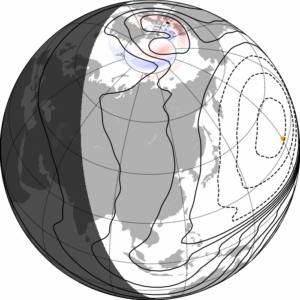# The average magnetic field and polar current system (AMPS) model

At about 10 times airline cruising altitude, at the boundary to space, the atmosphere is so thin that charged particles, electrons and ions, are not immediately neutralized in collisions. This inner edge of space, which extends from about 100 to 1000 km altitude, is called the ionosphere. Unlike the neutral atmosphere, the charged particles feel the forces of electromagnetism. These forces depend strongly on the interaction between the solar wind and the Earth’s magnetic field, which happens much further out, at about 10 Earth radii (roughly twice as distant as geostationary satellites, but well inside lunar orbit). The result of this interaction is communicated to the upper atmosphere along magnetic field lines, and as a result, a 3-dimensional current system is generated in the ionosphere. This current system can change very rapidly, partly because of changes in the solar wind and in the interplanetary magnetic field.The animation shows one day of AMPS model ionospheric currents (credit: KM Laundal, BCSS)

The AMPS model describes the average ionospheric magnetic field and current system for a given solar wind speed, interplanetary magnetic field vector, orientation of the Earth’s magnetic dipole axis, and the value of a solar flux index (F10.7). The model is empirical, which means that it is derived from measurements. We use measurements of the magnetic field, from the CHAMP and Swarm satellites, in low Earth orbit. For each measurement, model estimates of Earth’s own magnetic field, and the magnetic field associated with large-scale magnetospheric currents, have been subtracted. The remaining magnetic field is assumed to be related to ionospheric currents. Millions of measured magnetic field vectors are used to fit several thousand unknown parameters in an intricate mathematical function that describes the ionospheric magnetic field at any point in time and space. Electric currents are calculated from the magnetic field.

Model values of AMPS magnetic fields and currents can be calculated with the Python library pyAMPS, which is located here:

https://github.com/klaundal/pyAMPS